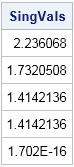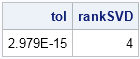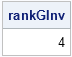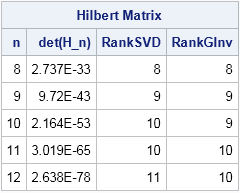A common question from statistical programmers is how to compute the rank of a matrix in SAS. Recall that the rank of a matrix is defined as the number of linearly independent columns in the matrix. (Equivalently, the number of linearly independent rows.) This article describes how to compute the rank of a matrix in SAS by using functions in SAS/IML software.

An important application of the rank is to determine whether a square matrix is nonsingular. The techniques in this article can also help you decide whether a square matrix is singular.

The rank of a matrix is one of those vexing problems in numerical linear algebra. Mathematically, the question is not difficult. However, in finite-precision arithmetic, computing the rank of a matrix (and whether it is nonsingular) can be challenging. This article suggests some best practices. The details have filled many books, journal articles, and PhD dissertations.

### The mathematical rank of a matrix

In theory, you can use Gaussian elimination to compute the rank of a matrix. You reduce the matrix to row echelon form; the rank is the number of rows that contain a nonzero element.

For square matrices, the same mathematical process determines whether a matrix is nonsingular. Other mathematical facts are also true: a singular matrix has a determinant equal to 0, at least one eigenvalue that is 0, and at least one singular value that is 0.

However, these mathematical facts are not good ways to numerically determine whether a matrix is rank-deficient or singular. A naive implementation of Gaussian elimination is numerically unstable. A numerical determinant that should be mathematically zero might be computed as a very tiny nonzero number in finite-precision arithmetic. Or an ill-conditioned but non-singular matrix might have a determinant so small that it is numerically indistinguishable from a singular matrix. In any case, you should never compare a numerical value to 0.

### The numerical rank of a matrix

So what's a statistical programmer to do? For the rank problem, there are several numerically robust techniques, but I will describe two that are based on the singular value decomposition (SVD). Some researchers use methods based on the QR decomposition, which is faster to compute but less reliable.

Mathematically, the rank of a matrix equals the number of nonzero singular values. Therefore a simple algorithm to estimate the rank is to use the SVD routine in SAS/IML to compute the singular values and then declare that any singular value smaller than some cutoff value is numerically the same as zero. The following example computes and displays the singular values for a 6x5 matrix by using the SVD:

```proc iml; /* rank(A)=4 because only four linearly independent columns */ A = {1 0 1 0 0, 1 0 0 1 0, 1 0 0 0 1, 0 1 1 0 0, 0 1 0 1 0, 0 1 0 0 1 };   call svd(U, Q, V, A); print Q[L="SingVals"];```You can see that the last singular value is tiny, and in fact the matrix is rank 4. Although you might be tempted to use a fixed cutoff value such as 1e-8 to determine which singular values are "small," it is usually better to incorporate factors that reflect the scale of the problem. A common cutoff value (used by MATLAB) is δ = d σmax ε where d is the maximum dimension of A, σmax is the maximum singular value of A, and ε is "machine epsilon." The machine epsilon can be computed by using the CONSTANT function in SAS. The following example computes the cutoff value and estimates the rank of the matrix:

```tol = max(dimension(A)) * constant("maceps") * (max(Q)); rankSVD = sum(Q > tol); print tol rankSVD;```There are other techniques that you can use to estimate the rank of a matrix. The SAS/IML documentation recommends using the generalized-inverse technique. The mathematical properties of the generalized inverse (also known as the Moore-Penrose inverse) are explained in a short 1978 article by M. James in The Mathematical Gazette.

The generalized inverse always exists, even for nonsquare matrices and for singular matrices. (If A is nonsingular, then the generalized inverse is the same as the inverse matrix A-1.) To reveal the rank of A, compute the trace of the product of the generalized inverse with A. For a nonsingular matrix, the trace is obviously the dimension of A. For nonsquare or singular matrices, the (rounded) trace is an estimate for the rank of the matrix (Penrose, 1954, p. 408), as follows:

```rankGInv = round(trace(ginv(A)*A)); print rankGInv;```The generalized-inverse technique does not enable you to specify a tolerance/scaling parameter, but a parameter is used internally by the GINV function to compute the generalized inverse.

It is useful to package up these techniques into a module, as follows:

```start RankMatrix(A, method="SVD", tol=); if upcase(method)="SVD" then do; call svd(U, Q, V, A); if IsEmpty(tol) then tol = max(dimension(A))*constant("maceps")*(max(Q)); return( sum(Q > tol) ); end; else do; return( round(trace(ginv(a)*a)) ); end; finish;   rankSVD = RankMatrix(A); rankGInv = RankMatrix(A, "GINV");```

### A numerical test for singularity

Let's test the rank algorithms on a notorious ill-conditioned matrix, the Hilbert matrix. For an n x n Hilbert matrix, the determinant approaches zero quickly, but is always positive, which means that the Hilbert matrix is nonsingular for all values of n.

The following table shows the result of computing the rank for five Hilbert matrices.The first column shows the size of the problem, which is also the true rank of the Hilbert matrix. The second column shows the determinant of the matrix, which shows that the matrices are very close to being singular. The third column shows the estimated rank by using the SVD algorithm. The SVD algorithm performs very well. It correctly computes the rank of the Hilbert matrix for n ≤ 10, and correctly finds the rank of a matrix with determinant 2E-53. The fourth column shows the estimated rank by using the generalized inverse algorithm. It also performs well and correctly computes the rank of the Hilbert matrix for n ≤ 9 and for a determinant as tiny as 1E-42.

In summary, both methods perform well. The SVD method is slightly faster and performed better on this test, so I recommend that you use the SVD method to estimate the rank of rectangular matrices and to determine whether a square matrix is nonsingular. The SVD method is the default method for the RankMatrix function.

ShareDistinguished Researcher in Computational Statistics

Rick Wicklin, PhD, is a distinguished researcher in computational statistics at SAS and is a principal developer of SAS/IML software. His areas of expertise include computational statistics, simulation, statistical graphics, and modern methods in statistical data analysis. Rick is author of the books Statistical Programming with SAS/IML Software and Simulating Data with SAS.

1.Rick,
I find a better function to get rank of a matrix. It can get exact n=16 rank of Hilbert Matrix .

proc iml;
n=20;
start Hilbert(n);
i = repeat(T(1:n), 1, n); /* = row(H) */
j = repeat(1:n, n); /* = col(H) */
return( 1 / (i + j - 1) );
finish;
H = Hilbert(n);
x=ECHELON(H);
print x;

•Yes, I discuss Gaussian elimination (which is what the ECHELON function uses) in the first subsection of this article.